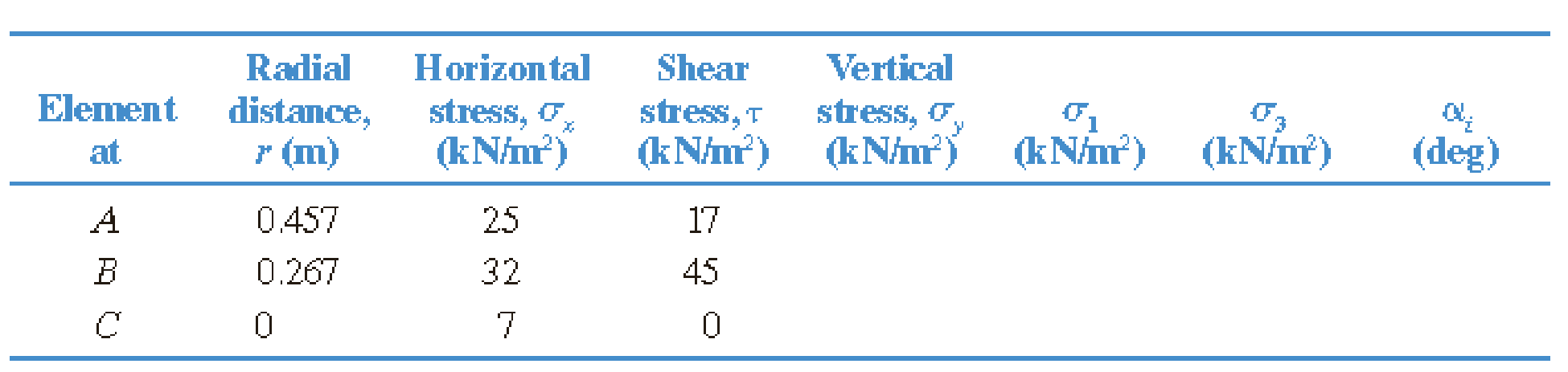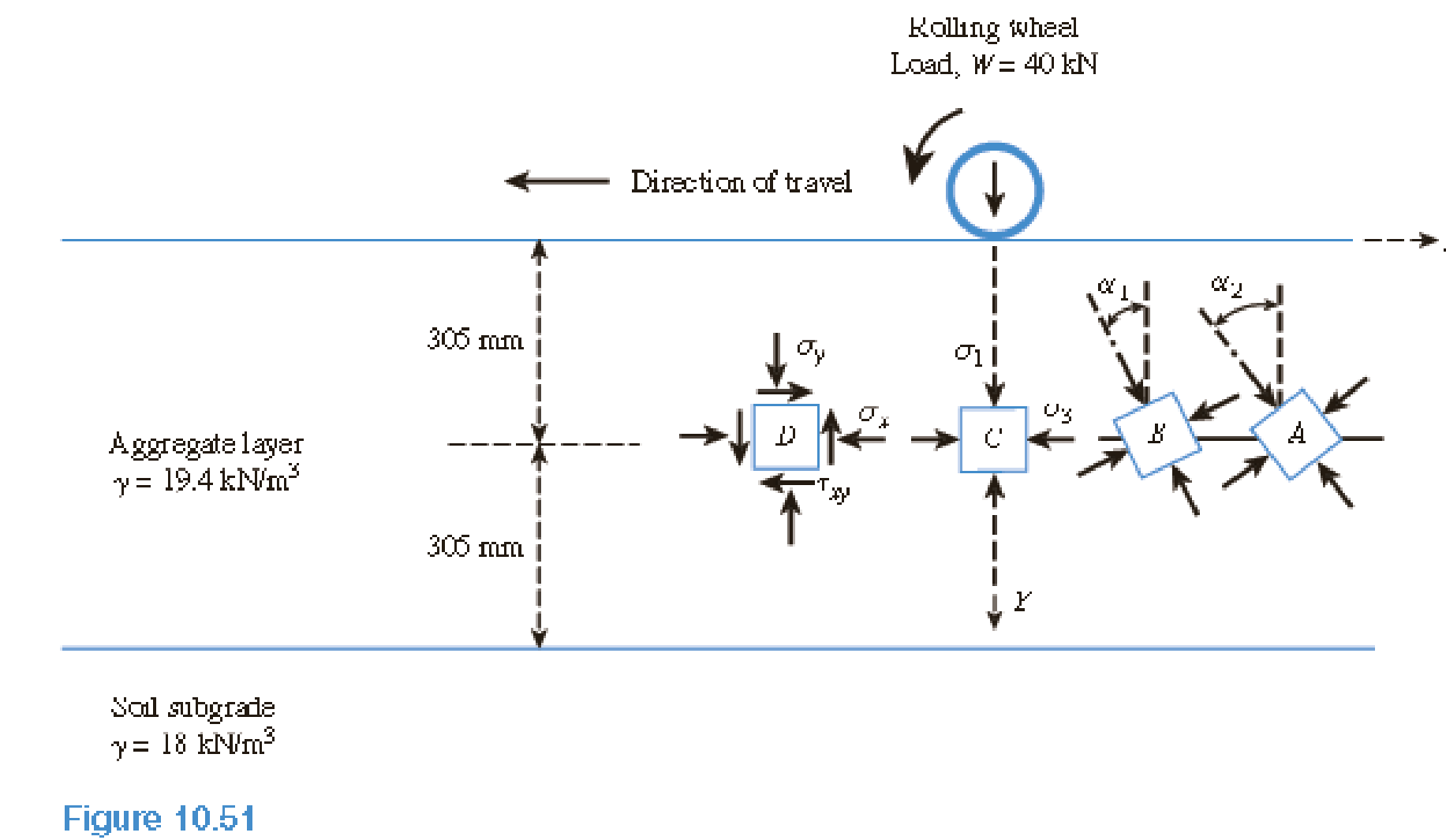Chapter 10, Problem 10.2CTPPrinciples of Geotechnical Enginee...

9th Edition
Braja M. Das + 1 other
ISBN: 9781305970939

Solutions

Chapter
SectionPrinciples of Geotechnical Enginee...

9th Edition
Braja M. Das + 1 other
ISBN: 9781305970939
Textbook Problem

A soil element beneath a pave ment experiences principal stress rotations when the wheel load, W, passes over it and moves away, as shown in Figure 10.51. In this case, the wheel load has passed over points A and B and is now over point C. The general state of stress at these points is similar to the one shown by a stress block at point D. The phenomenon of principal stress rotation influences the permanent deformation behavior of the pavement layers.Investigate how the magnitude and the orientations of the principal stresses vary with distance from the point of application of the wheel load. Consider the case shown in Figure 10.51. An unpaved aggregate road with a thickness of 610 mm and unit weight of 19.4 kN/m3 is placed over a soil subgrade. A typical single-axle wheel load, W = 40 kN, is applied uniformly over a circular contact area with a radius of R = 150 mm (tire pressure of 565 kN/m2). The horizontal and shear stresses at each point are calculated from a linear elastic finite element analysis for a two-layer pavement and are presented in the following table.a. Use Eq. (10.28) to calculate the vertical stress increases at soil elements A, B, and C that are located at radial distances 0.457,0.267, and 0 m, respectively, from the center of the load. Determine the total vertical stress (σy) due to wheel load, the overburden pressure at each point, and enter these values in the table. b. Use the pole method to determine the maximum and minimum principal stresses (σ1 and σ3) for elements A, B, and C. Also determine the orientation (αs) of the principal stress with respect to the vertical. Enter these values in the table. c. Plot the variations of σ1 and αs, with normalized radial distance, r/R, from the center of loading.

(a)

To determine

Calculate the total vertical stress (σy) due to wheel load and the over burden pressure at each point.

Explanation

Given information:

The thickness of unpaved aggregate road (t) is 610mm.

The unit weight of aggregate layer (γagg) is 19.4kN/m3.

The radius of the wheel (R) is 150mm.

A typical single-axle wheel load (W) is 40kN.

The tire pressure (q) is 565kN/m2.

The radial distance, horizontal stress, and shear stresses at each point are given in the Table.

Calculation:

Consider the unit weight of water (γw) is 9.81kN/m3.

Calculate the increase in vertical stress (Δσz) using the relation as follows.

Δσz=q(A+B) (1)

Here, A and B are the factors and a functions of zR and rR.

Calculate the depth to radius ratio (zR) as shown below.

Substitute 305mm for z and 150mm for R.

zR=305150=2.032

For the radial distance (r) of 0.457m:

Calculate the ratio (rR) as shown below.

Substitute 0.457m for r and 0.150m for R.

rR=0.4570.150=3.053

Similarly calculate the remaining values and tabulate as in Table 1.

Calculate the value of A as shown below.

Refer Table 10.8 “Variation of A with zR and rR” in the Text Book.

Take the value of A as 0.02221, for the values zR of 2 and rR of 3.

Similarly calculate the remaining values and tabulate as in Table 1.

Calculate the value of B as shown below.

Refer Table 10.9 “Variation of B with zR and rR” in the Text Book.

Take the value of B as 0.00028, for the values zR of 2 and rR of 3

(b)

To determine

Calculate the maximum and minimum principal stresses for elements A, B, and C and the orientation of the principal stress with respect to the vertical using pole method.

(c)

To determine

Plot the variations of σ1 and     αi with normalized radial distance rR, from the center of loading.

Still sussing out bartleby?

Check out a sample textbook solution.

See a sample solution

The Solution to Your Study Problems

Bartleby provides explanations to thousands of textbook problems written by our experts, many with advanced degrees!

Get Started

What is structural independence, and why is it important?

Database Systems: Design, Implementation, & Management

What is the purpose of layout fluid (dye)?

Precision Machining Technology (MindTap Course List)

What is the difference between a detail report, a summary report, and an exception report?

Systems Analysis and Design (Shelly Cashman Series) (MindTap Course List)

List characteristics of a smartphone.

Enhanced Discovering Computers 2017 (Shelly Cashman Series) (MindTap Course List)

What should be done with a leaking cylinder if the leak cannot be stopped?

Welding: Principles and Applications (MindTap Course List)